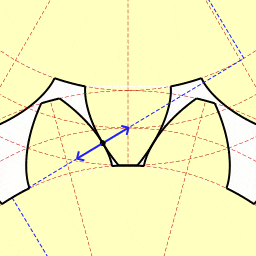# Action-Reaction forces and angles

• B
Sundown444
Now, I am sure we all know that objects and their action-reaction forces happen in terms of exerting forces at opposite directions but with the same magnitude. That said, I wish to ask: Do objects with action-reaction forces have their forces exerted in opposite angles as well as opposite directions?

2022 Award
What do you mean by "opposite angles"? I can't see what you could mean by this that isn't covered by "opposite direction".

•Dale
Sundown444
Or I guess I am asking, more precisely, do objects with action-reaction forces have act be exerted at different angles from each other, or the same?

2022 Award
I still don't understand. Can you give an example of two things that are in opposite directions but not opposite angles, however you are defining this?

Sundown444
Say a ball is hit by a ping pong paddle. The paddle hits the ball at 45 degrees. But the top of the ball is hit, say at 90 degrees. Would the angles between these two objects not be opposite in angles, even though the parts pushing each other are the same direction?

Mentor
Say a ball is hit by a ping pong paddle. The paddle hits the ball at 45 degrees. But the top of the ball is hit, say at 90 degrees. Would the angles between these two objects not be opposite in angles, even though the parts pushing each other are the same direction?
The direction that the ball hits the paddle or the paddle hits the ball is not relevant. What is relevant is the direction of the force. It is the direction of the two forces that are equal and opposite. The angle of the ball doesn't enter into it at all.

•Ibix
Sundown444
So the angle does not matter in action reaction forces, you say?

Mentor
So the angle does not matter in action reaction forces, you say?
The angle of the force matters. The angle of the ball or the paddle or whatever doesn't matter except insofar as it affects the angle of the forces

Sundown444
So, are the angles of the force opposite, or same, or different to each other?

So, are the angles of the force opposite, or same, or different to each other?
The angle between the 3rd law forces is 180°.

•Sundown444 and Lnewqban
Sundown444
The angle between the 3rd law forces is 180°.

Okay, just wanted to make sure. Thanks!

Homework Helper
Gold Member
... Do objects with action-reaction forces have their forces exerted in opposite angles as well as opposite directions?
The angle formed between both force vectors must be always π, otherwise we will have a third resulting vector force.•Sundown444